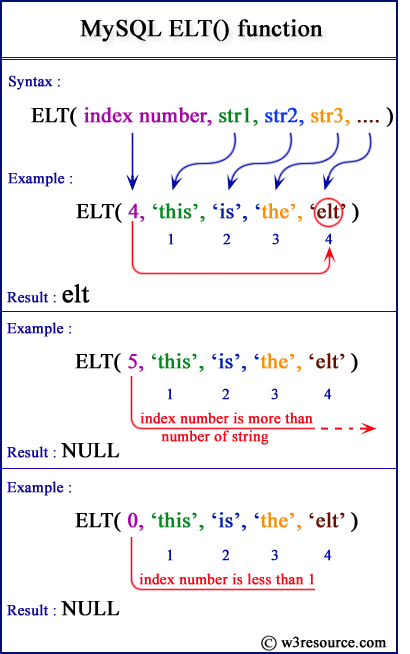# MySQL ELT() function

## ELT() function

MySQL ELT() returns the string at the index number specified in the list of arguments. The first argument indicates the index of the string to be retrieved from the list of arguments.

It returns NULL when the index number is less than 1 or index number is greater than the number of the string specified as arguments.

Note: According to Wikipedia ELT stands for Extract, Load, Transform (ELT), a data manipulation process.

Syntax:

```ELT(index number, string1, string2, string3,…)
```

Argument:

Name Description
index number An integer.
string1, string2, string3,… List of strings.

MySQL Version: 5.6

Video Presentation:

Pictorial PresentationExample: MySQL ELT() function

The following MySQL statement will return the string at 4th position from the list of strings.

Code:

``````SELECT ELT(4,'this','is','the','elt');
```
```

Sample Output:

```mysql> SELECT ELT(4,'this','is','the','elt');
+--------------------------------+
| ELT(4,'this','is','the','elt') |
+--------------------------------+
| elt                            |
+--------------------------------+
1 row in set (0.02 sec)
```

Example of MySQL ELT() function with greater index number

The following MySQL statement will return NULL because the index number is more than the number of strings.

Code:

``````SELECT ELT(5,'this','is','the','elt');
```
```

Sample Output:

```mysql> SELECT ELT(5,'this','is','the','elt');
+--------------------------------+
| ELT(5,'this','is','the','elt') |
+--------------------------------+
| NULL                           |
+--------------------------------+
1 row in set (0.00 sec)
```

Example of MySQL ELT() function with index value zero(0)

The following MySQL statement will return the NULL because the index number is less than 1.

Code:

``````SELECT ELT(0,'this','is','the','elt');
```
```

Sample Output:

```mysql> SELECT ELT(0,'this','is','the','elt');
+--------------------------------+
| ELT(0,'this','is','the','elt') |
+--------------------------------+
| NULL                           |
+--------------------------------+
1 row in set (0.00 sec)
```

All String FunctionsPrevious: CONCAT
Next: EXPORT_SET

﻿